Instantly share code, notes, and snippets.

# hhyyg/operators.md

Last active May 8, 2019 17:23

# A add: Outputs the sum of inputs.

``````aAb
z
``````
``````z = a + b
``````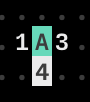# B bounce: Bounces between two values based on the runtime frame.

``````rBt
z
``````

# C clock: Outputs a constant value based on the runtime frame.

``````xCy
z
``````
• x: rate
• y: mod
``````// 1frame : 1x
for (i = 0; i++; i < y) {
z = i;
}
``````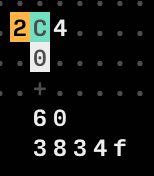# D delay: Bangs on a fraction of the runtime frame.

``````xDy
z
``````
• x: rate
• y: mod

1 bangs / x * y frame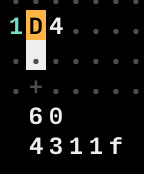-----> E ----->

# F if: Bangs if both inputs are equal.

``````aFb
z
``````
``````if (a == b) {
z = Bangs;
}
``````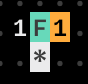# G generator: Writes distant operators with offset.

``````xylG
z
``````
• x: offset x
• y: offset y
• l: length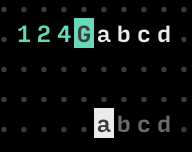# H halt: Stops southward operators from operating.

``````H
* #<-- stop
``````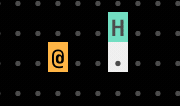# I increment: Increments southward operator.

``````xIy
z
``````
• x: min
• y: max
``````for (i = x; i++; i < y) {
z = i;
}
``````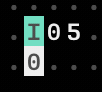# J jumper: Outputs the northward operator.

``````x
J
z
``````
``````z = x;
``````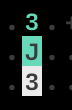# K konkat: Outputs multiple variables.

It works with variables.

``````lK[variables...]
[z]
``````

l:length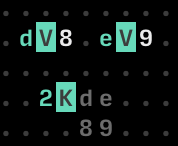# L loop: Loops a number of eastward operators.

``````rlL[...z]
``````

r: rate l: length

# M modulo: Outputs the modulo of input.

``````aMb
z
``````
``````z = a % b
``````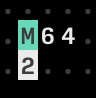# N north: Moves Northward, or bangs.

``````↑
N
``````

# O offset: Reads a distant operator with offset.

``````xyOp
z
``````
• x: offset x
• y: offset y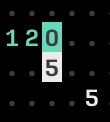# P push: Writes an eastward operator with offset.

``````xyPi
z
``````
• x: offset
• y: length(capacity size of offset)
• i: input value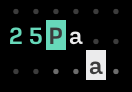# Q query: Reads distant operators with offset.

``````xylQp
z
``````
• x: offset x
• y: offset y
• l: length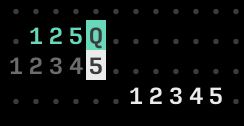# R random: Outputs a random value.

``````xRy
z
``````
• x: min value
• y: max value
• z = Random(x..<y)

# S south: Moves southward, or bangs.

``````S
↓
``````

# T track: Reads an eastward operator with offset.

``````xyT[input values]
z
``````
• x: key
• y: length
• z = (input values)[key]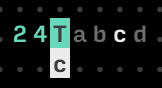# U uturn: Reverses movement of inputs.

U like a mirror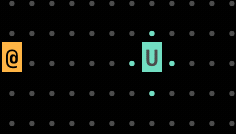# V variable: Reads and write globally available variables.

Write:

``````aV2
``````
``````let a = 2
``````

`````` Va
z
``````
``````z = a
``````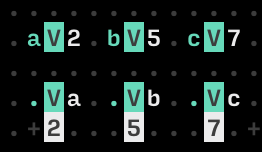<--- W

# X teleport: Writes a distant operator with offset.

``````xyXv
z
``````
• x: offset x
• y: offset y
• v: input value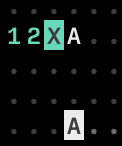# Y jymper: Outputs the westward operator.

``````xYz
``````
``````z = x
``````

# Notes

Move operators:

``````.N.
W.E
S.
``````
``````Z
``````
This file contains bidirectional Unicode text that may be interpreted or compiled differently than what appears below. To review, open the file in an editor that reveals hidden Unicode characters. Learn more about bidirectional Unicode characters
 ................................................................. ............................................3.................... .A12.0B.1C4.2D3..F33..124Gabcd...H..I2b.....J............3L312... .3.......3.......*..................5.......3.................... ................................................................. ..........................abcd................................... ................................................................. ................................................................. .....M65..12O...25Pa.....125Q...........R2a.......#NS............ .....1......5.......a...12345...........5........#W.E............ ..............5...............12345...............#S............. ................................................................. ................................................................. ..13Tabc......U...00Xz...4Y4....................Z................ ....b...............z.............Z....Z......................... ................................................................. ................................................................. ................................................................. ..............................dV8.eV9............................ ................................................................. ...............................2Kde.............................. ....aV2.bV5.cV7..................89.............................. ................................................................. .....Va..Vb..Vc.................................................. .....2...5...7...................................................